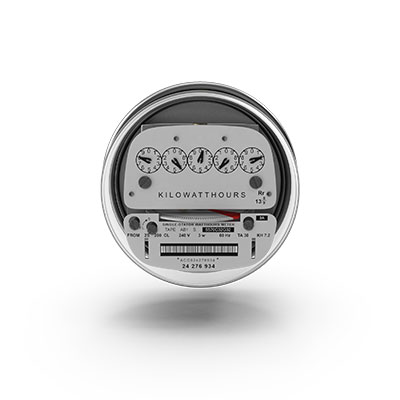#### Reading Your Electric Meter

Your electric bill is calculated in kilowatt-hours or the number of kilowatts of power times hours of use in the monthly billing period. By reading your meter at the same time each day, you will get an exact total of the electricity you have used. By writing each daily total on your Energy Diary, you can chart increases and decreases in your energy use. By noting high consumption activity, such as laundering or cooking, you will know how you are spending your energy dollar.Some meters are digital and reading them is simple. All you do is look at the numbers on the face and note the reading. Others meters operate using gears to turn dials. These take a little more thought to read correctly.

Notice that some of the dial hands on the meter above turn "clockwise" and some turn "counter-clockwise". Look at the first dial, it is turning "clockwise", and the hand is between the "7" and the "8". Record the number that the hand just passed - (in this case "7").

The second dial is turning "counter-clockwise" and is just past the "3", so that is the next number to write down and so on.

Look at the dial to the right of the dial being "read". If the hand on the right has moved past "zero", write down the number the hand on the left is pointing to-(using the same dials it would be the "7").If the hand on the right is not past "zero", then write down the next lowest number on the dial being "read". In this illustration, the meter reads "73256" at this time.

Now, if you read this meter at the same time tomorrow, subtract tomorrow's "reading" from today's "reading" to determine the number of kilowatt-hours used in one day.

To calculate the cost of the energy use, multiply the number of kilowatt-hours by the cost per kilowatt-hour charged by your energy supplier. For example, at the national average of 9 cents multiply by \$.09.

Remember, just reading a meter alone will not save money; however, it will help you become more aware of overall energy consumption, and being aware is the first step to making wise decisions.